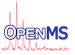OpenMS  2.8.0
SignalToNoiseEstimatorMedianRapid Class Reference

Estimates the signal/noise (S/N) ratio of each data point in a scan by using the median (window based) More...

`#include <OpenMS/FILTERING/NOISEESTIMATION/SignalToNoiseEstimatorMedianRapid.h>`

Collaboration diagram for SignalToNoiseEstimatorMedianRapid:
[legend]

## Classes

struct  NoiseEstimator
Class to compute the noise value at a given position. More...

## Public Member Functions

SignalToNoiseEstimatorMedianRapid (double window_length)
default constructor More...

NoiseEstimator estimateNoise (OpenMS::Interfaces::SpectrumPtr spectrum)
Compute noise estimator for an m/z and intensity array using windows. More...

NoiseEstimator estimateNoise (OpenMS::Interfaces::ChromatogramPtr chrom)
Compute noise estimator for an m/z and intensity array using windows. More...

NoiseEstimator estimateNoise (const std::vector< double > &mz_array, const std::vector< double > &int_array)
Compute noise estimator for an m/z and intensity array using windows. More...

## Private Member Functions

void computeNoiseInWindows_ (const std::vector< double > &mz_array, std::vector< double > int_array, std::vector< double > &result, double mz_start)
Computes the noise in windows for two input arrays and stores the median intensity in the result (internal) More...

double computeMedian_ (std::vector< double >::iterator &first, std::vector< double >::iterator &last)
Median computation on a part of an array [first,last) More...

## Private Attributes

double window_length_
Window length parameter. More...

## Detailed Description

Estimates the signal/noise (S/N) ratio of each data point in a scan by using the median (window based)

For each scan, we define a set of windows of a pre-defined size (param: window_length_) in m/z domain for which the intensity median is calculated. The noise for a data point is estimated to be the median of the intensities of the current window.

To get a more robust noise estimate, the nose value is calculated two times for two sets of windows that are shifted by 1/2 of the window size and the reported noise value is the average of the two.

A call to estimateNoise will return an object of type NoiseEstimator which then provides a function get_noise_value which will return the noise value for a given m/z value.

The idea behind this class is to have an estimator for signal to noise that gives similar results to SignalToNoiseEstimatorMedian but performs faster. Note that it will not give identical results as SignalToNoiseEstimatorMedian but for many application the results from this class will be sufficient.

## ◆ SignalToNoiseEstimatorMedianRapid()

 SignalToNoiseEstimatorMedianRapid ( double window_length )
inline

default constructor

## ◆ computeMedian_()

 double computeMedian_ ( std::vector< double >::iterator & first, std::vector< double >::iterator & last )
private

Median computation on a part of an array [first,last)

Note
Does not guarantee that the elements between [first, last) are in the same order as before (they most likely will not be).

## ◆ computeNoiseInWindows_()

 void computeNoiseInWindows_ ( const std::vector< double > & mz_array, std::vector< double > int_array, std::vector< double > & result, double mz_start )
private

Computes the noise in windows for two input arrays and stores the median intensity in the result (internal)

Note that int_array is copied on purpose, since it is modified while sorting, a copy is needed.

## ◆ estimateNoise() [1/3]

 NoiseEstimator estimateNoise ( const std::vector< double > & mz_array, const std::vector< double > & int_array )
inline

Compute noise estimator for an m/z and intensity array using windows.

Will return a noise estimator object.

## ◆ estimateNoise() [2/3]

 NoiseEstimator estimateNoise ( OpenMS::Interfaces::ChromatogramPtr chrom )
inline

Compute noise estimator for an m/z and intensity array using windows.

Will return a noise estimator object.

## ◆ estimateNoise() [3/3]

 NoiseEstimator estimateNoise ( OpenMS::Interfaces::SpectrumPtr spectrum )
inline

Compute noise estimator for an m/z and intensity array using windows.

Will return a noise estimator object.

## ◆ window_length_

 double window_length_
private

Window length parameter.